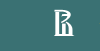Раскрыть

Armyanskii ln., 4-2, Moscow, 101000, Russia

Е-mail:
hsejournal.psychology@gmail.com

## The Role of Mathematical Psychology in Social Sciences

2014. Vol. 11. No. 3. P. 8–22 [issue contents]

The article raises the question of the role of mathematics in the social sciences, describes the stages and steps of mathematization of psychological knowledge. The paper describes the mathematization of psychology in the narrow (use of formal mathematical language to describe mental phenomena and processes) and broad sense (adoption of natural science tradition of logical rigor and scientific thinking). The object, subject and method of mathematical psychology are introduced. The main method of mathematical psychology is modelling. The article presents a classification of mathematical models by mathematical apparatus: stochastic, deterministic and synergetic models. A brief description of the significant models of psychic reality, emphasizing models developed in Russia, is provided. The paper describes the current state of mathematical psychology and argues that it is characterized by not only using new mathematical principles, models, and methods, but also new understanding of existing approaches. At the moment, the analysis of the dynamics of mental processes, individual differences, the structure of the personality and interpersonal interaction becomes very important, so most probably, the synergetic approach, soft computing, quality integration and asymptotic mathematics should be considered as the most promising techniques for the modelling of mental systems, the interaction dynamics, the learning process and self-learning systems. There is a need in new approaches to psychological measurement, modelling of behavioural macro-dynamics as a result of micro-dynamic processes in the human psyche and modelling of natural systems (management, psychotherapy).To achieve this, psychologists would require a deeper mathematical understanding. Mathematical courses should be based on competence approach and take into account the demands of practice. Natural-scientific training of social sciences students should take into account the present state of mathematical psychology. Mathematical disciplines should help students to think outside the box, understand the logic of irrational behaviour, find creative solutions and not be limited by the formal use of mathematical methods as part of data processing.

Citation: Savchenko, T.N., & Golovina, G. M. (2014). The Role of Mathematical Psychology in Social Sciences. Psychology. Journal of Higher School of Economics, 11(3), 8-22 (in Russian)
BiBTeX
RIS